Internet of Things and Cloud Computing
Volume 3, Issue 3, October 2015, Pages: 29-32

Building Multile Multicast Trees with Guarranteed QOS for Service Based Routing Using Artificial Algorithms

Nguyen Thanh Long1, Nguyen Duc Thuy2, Pham Huy Hoang3

1Software development division III, Informatics Center of Hanoi Telecommunications, Hoan Kiem, Hanoi, Vietnam

2Center for applied research and technology development, Research institute of Posts and telecommunications, Hanoi, VietNam

3Information technology institute, Ha Noi University of Science Technology, Hanoi, Vietnam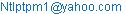(N. T. Long)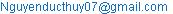(N. D. Thuy)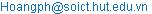(P. H. Hoang)

Nguyen Thanh Long, Nguyen Duc Thuy, Pham Huy Hoang. Building Multile Multicast Trees with Guarranteed QOS for Service Based Routing Using Artificial Algorithms. Internet of Things and Cloud Computing. Vol. 3, No. 3, 2015, pp. 29-32. doi: 10.11648/j.iotcc.s.2015030601.13

Abstract: In Service Based Routing (SBR), data is transmitted from a source node to destination nodes are not depended on destination addresses. Hence, it is comfortable with new advanced technology as cloud computing and also flexible and reliable. Multicast routing is advanced technique to deliver data simultaneously from one source node to multiple destination nodes with QOS (quality of service). In this paper, we introduce a technique that is extended from multicast technique with multiple multicast trees that are conformed quality of service routing. This technique is based on Greedy, Ant Colony Optimization, and fuzzy logic to get optimal routes to transmit data from one source to multiple destination node very effectively. The usage of the ANT Colony optimization, Greedy, fuzzy logic algorithms to find cyclic or multiple paths routes on each trunk by multiple criterions to transmit data effectively.

Keywords: MANET, Service, Routing, Multi-Paths, Bandwidth, Cluster, ANT, Tree, Multicast, Colony, Optimization, Greedy, QOS, MST

1. Basical Concepts

In order to model a general network in common by a graph in discrete mathematics. In which denote V is the set of vertics that are nodes in the network, E is the set of the edges that are links to connect each pair of nodes in the graph. The routing problem is to make optimization routes from routing table. The inputs of the routing table are collected by routing process. The routing process consists of several minor detail processes: In the reactive or on-demand routing protocol: i) Broadcast packets over network to find routes; ii) Collecting reply packets to build optimal routes; in proactive protocol it usually collecting network information through flooding HELLO messages. On receiving HELLO packets, network node updates routing tables to use to find routes as introducing in the next section. HELLO messages are effective to collect network information.

2. Artificial Algorithms

2.1. Fuzzy Logic

The fuzzy logic  is mathematic logical method based on the probabilistic of the related factors. We all know that in reality every thing also has a level of true, especially in MANET, all nodes continuous move with changing velocity and very limited energy. So applying this kind of logic to assess the metrics of MANET is very comfortable. For example, GOOGLE are building many automatically system to coordinate vehicle system such as satellite system, planet, astronaut, so every thing can be apply this theory. So MANET routing is granted an important role in these systems. In the moving using GREEDY and ACO algorithms is very comfortable to assess the velocity and orbital motion of moving objects. In particular, the coordination of moving system can be tracked by GPS system and these can be put into artificial system to optimize. IOT is short for Internet of thing that is a trend to connect all the things of the Planet. So from living tools to astronaut all connected by Global Internet. For example, on the move we can connect to operate our work.

2.2. Greedy Algorithm

The greedy algorithm is very popular in vehicle routing. That may be effective in MANET routing because in this routing all nodes are usually to move randomly. So in this paper will focus on these algorithms to make multiple paths routing with quality of service . The Greedy algorithm executes based on heuristic principle, that continuously find local optimal solution in each step of the total operation until global optimal solution found or a predefined processing steps.

2.3. Ant Colony Optimization

The ACO and Greedy algorithms are two artificial algorithms, which choose routes based on probability of each connection between each pair of nodes. The probability of each connection is assigned by fuzzy logic introduced above. When the detecting process operates, that assigns each connection found a weight. This weight is used to calculated probability for choosing the route. The probability may be calculates by some methods, for example using RREQ messages that emitting from a node and the replied RREP messages. RREP contains information to measure connection probability. Beside, in proactive routing, it is based on nodes’ received HELLOs information to assess probability of connection: Prob(Connection(i, j))=F(M_1, M_2,…, M_n), M_1, M2, …, M_i are some metrics to assess connection.

Prob(Route)=∏(Prob(Connection(i, j)))|, (i, j) is connection of this route.

3. Build Multicast Tree

3.1. Use Greedy Algorithm

3.1.1. Find Minimum Spanning Tree (MST)

i) Using three above algorithms [1-2] to find and assign weight for each edge of the graph.

Cost(E(v_1, v_2))= FZY|GRD|ACO(E(v_1, v_2))    (1)

ii) At first making minimum spanning tree, then regulating this tree to get multicast tree. Sorting the edges of the graph descending;

iii) Remove edge beginning from the first element of this sorted list individually with the condition that this process doesn’t dividing this graph into two disjoint components;

iv) Check the number of edges of the graph, if it is equal to number of vertices minus one. If the condition is true then the algorithm ends to get the tree.

Count(edges) = Count(vertices) - 1.         (2)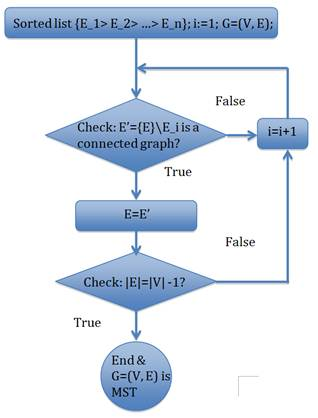Figure 1. The flow diagram of algorithm to find a MST tree.

3.1.2. Find Multicast Tree

We denote multicast tree by: (S, {D_1, D_2, …, D_n}), s is source node, D={D_1, D_2, …, D_n} is destination set. Choose the root of the tree, which is the source node to the tree, D={Ø}, edges scanned set SC={Ø}. Tracing the tree from this source node to the destination nodes individually by all directions following the edges that are not in SC: S à {C_1, C_2, …, C_n}. For each C_i: i) check whether C_i is in SC, if not: ii) check whether C_i is destination node, if true: D=D È C_i; iii) SC=SC È (S, C_i); iv) scan C_i by above i), ii), iii) steps. To each destination node, if it continues connecting to another nodes, using GEN/ BEE/ ACO algorithms to find optimal path for remaining nodes. Otherwise using the next steps to get the optimal solution.

The alg. ends when all destination nodes are added to the tree. All branches of the tree that don’t end with a destination node are being cut.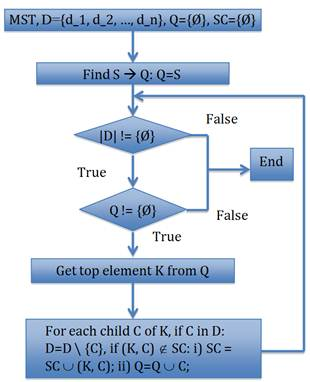Figure 2. The diagram of algorithm to make a multicast tree from MST.

3.1.3. Assessing this Algorithm

The MST finding algorithm MST has complexity nearly to O(log(|V|)*(|S(edges)|-|V|)).

3.2. Use KrusKal Algorithm

This algorithm picks edges for MST depends on the principle:

i) Sort the edge set in ascending order: {E_1, E_2, …, E_n}.

ii) Make for each vertex v a set of vertices V that are all connected to V. At first assign: V={v}. Assume i is the current edge picked, E_i=(v_k, v_h), if v_k and v_h are belonged to two disjoint sets of vertices (that have no common vertices: V_k Ç V_h = Æ), add E_i to the MST, We update:

MST = MST È E_i, merge V_k and V_h into V_k:

V_k = V_k È V_h.                   (3)

Until the number of elements of MST equal to |V| - 1.

At that time all vertices are in common one connected graph with total minimum distance between these vertices. That also means all disjoint vertices sets are merged into one component. The complexity of this algorithm is less than above introduced algorithm. Because the complexity of this algorithms is reduced after each round. The number of disjoint sets is reduced by one after a edge is added to the MST. Only when number of element of MST is equal to number of vertices minus one then the algorithm ends:

O(Alg.) = |V| * (|V|-1) / 2.           (4)

So it is very good for the network with number of nodes is not large.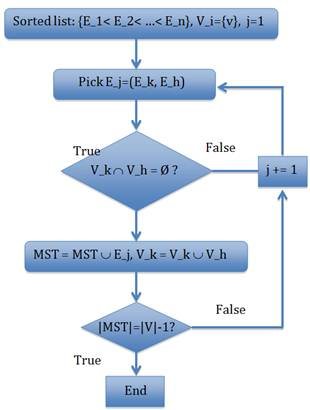Figure 3. The flow diagram of Kruskal algorithm to find a MST tree.

4. Make QOS Routes from Multiple Multicast Tree

We continuously apply the above algorithm to find some multicast trees. After finding out one tree, in the next step of finding using the edges minus all the edges of the found trees. So this algorithm converses fast. Until the remaining set of edges contains number of edges less than |Vertices|-1 or we can’t find out any solution then ending. Combining all found trees to get multiple path of each branch of the tree to make QOS routes.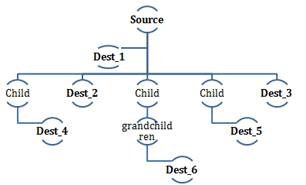Figure 4. The multicast tree from a source node to six destination nodes.

When joining found multicast trees, denote a multicast MST(S, S(D)), S is the source node, S(D) is the set of the destination nodes. Combined multicast tree is denoted by: MBT(S, S(D)).

MBT(S, S(D)) = Combine(MST(S, S(D)))    (5)

So each route is multiplied by combining some gradients paths from these MST trees. So the bandwidth of route is easily to increase to meet the demand.

5. Build Hierachical Multiple Multicast Routing

In the papers [1-2-6-7] we have mentioned some strategies to make hierarchical routing. In the global network, applying the R+ tree to manage the network. Assume, at a level in this tree, R is the vertex at this level, this vertex has n child vertices {C_1, C_2, …, C_n}, which are R+ tree children of their parent. So we have:

R+= R+(C_1) È R+(C_2) ÈÈR+(C_n) (6)

In which, C_i is root of a child R+ tree, in each child R+ tree, we use the introduced algorithms to make multiple multicast trees to route in this cluster of whole network. In each multicast tree, it may be used an optimal algorithm such as GEN or BEE or ACO [1-2-3-7-8] to find optimal routes for data transmission.

6. Conclusions

Normal network node can use proactive or reactive or on-demand routing protocol based on network situation and mobile rate of nodes. So applying above algorithms to find multiple paths routes with QOS guaranteed is very effectively and the capability to scale to large networks. When the number of nodes increases, we may use the above-introduced R+ tree to make hierarchical multicast routing. The purpose of hierarchical multicast routing is mainly aimed to reduce overhead in large network routing. The algorithms that are used for finding multiple multicast trees are both very comfortable for from small to large networks with guaranteed QOS.

References

1. Nguyen Thanh Long, Nguyen Duc Thuy, Pham Huy Hoang, Research on Applying Hierachical Clustered Based Routing Technique Using Artificial Intelligence Algorithms for Quality of Service of Service Based Routing, Internet of Things and Cloud Computing. Special Issue:Quality of Service of Service Based Routing. Vol. 3, No. 6-1, 2015, pp. 1-8. doi: 10.11648/j.iotcc.s.2015030601.11.
2. Nguyen Thanh Long, Nguyen Duc Thuy, Pham Huy Hoang, Research on Innovating and Applying Evolutionary Algorithms Based Hierarchical Clustering and Multiple Paths Routing for Guaranteed Quality of Service on Service Based Routing, Internet of Things and Cloud Computing. Special Issue:Quality of Service of Service Based Routing. Vol. 3, No. 6-1, 2015, pp. 9-15. doi: 10.11648/j.iotcc.s.2015030601.12.
3. Kartheek Srungaram, Dr. MHM Krishna Prasad, Department of Information Technology, JNTUK-UCEV, Vizianagaram, A.P, India, "ENHANCED CLUSTER BASED ROUTING PROTOCOL FOR MANETS".
4. Cândida Ferreira, Departamento de Ciências Agrárias, Universidade dos Açores, 9701-851 Terra-Chã, Angra do Heroísmo, Portugal, "Gene Expression Programming: A New Adaptive Algorithm for Solving Problems".
5. Bibhash Roy, Tripura Institute of Technology, Narsingarh, Tripura, India, "Ant Colony based Routing for Mobile Ad-Hoc Networks towards Improved Quality of Services".
6. Nguyen Thanh Long, Nguyen Duc Thuy, Pham Huy Hoang, "Innovating R Tree to Create Summary Filter for Message Forwarding Technique in Service-Based Routing", Springer, ISBN: 978-3-642-41773-3, LNICST 121, p. 178, 2013.
7. Research on Innovating, Applying Multiple Paths Routing Technique Based on Fuzzy Logic and Genetic Algorithm for Routing Messages in Service - Oriented Routing: Long Thanh Nguyen, Tam Nguyen The, Chien Tran, Thuy Nguyen Duc. Journal: Scalable Information Systems EAI.
8. A Particle Swarm Optimization with Adaptive Multi-Swarm Strategy for Capacitated Vehicle Routing Problem. Kui-Ting Chen, Ke Fan, Yijun Dai and Takaaki Baba, 1Research Center and Graduate School of Information, Production and Systems, Waseda University, 2-7 Hibikino, Kitakyushu, Fukuoka, Japan.
9. Z. Pooranian, A. Barati and A. Movaghar, "Queen-bee Algorithm for Energy Efficient Clusters in Wireless Sensor Networks", World Academy of Science, Engineering and Technology 49 2011.
10. Anjum A. Mohammed, Information Technology Department, College of Computer and Information Sciences,King Saud University, KSA, "Optimal Routing In Ad-Hoc Network Using GeneAlgorithm".
11. B.G.Obulla Reddy, Maligela Ussenaiah, Rayalaseema University, Andhra Pradesh, India, "An Adaptive Fuzzy Clustering and Location Management inMobile Ad Hoc Networks", International Journal of Computer Applications & Information TechnologyVol. I, Issue III, November 2012 (ISSN: 2278-7720).

 Contents 1. 2. 2.1. 2.2. 2.3. 3. 3.1. 3.2. 4. 5. 6.
Article ToolsAbstractPDF(289K)